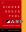Next Functional Programming in Perl 24

# Syntactic Difficulties

• Another example: Perl has a built-in map operator:

```        map { \$_ * 2 + 1 } 1..10;
```
• Suppose you want to write an analogous operator for an iterator

```        sub imap {
my (\$f, \$iterator) = @_;
return sub {
my \$next = \$iterator->();
return defined(\$next) ? \$f->(\$next) : undef;
}
}
```
• So far, so good

• But to call it, you must write

```        imap(sub { \$_ * 2 + 1 }, \$iterator);
```
• This kind of thing can be worked around through a variety of tricks

• You can in fact squeeze out this syntax:

```        imap { \$_ * 2 + 1 } \$iterator;
```

 NextCopyright 2005 M. J. Dominus